# KSEEB Solutions for Class 10 Science Chapter 1 Chemical Reactions and Equations

Students can Download Science Chapter 1 Chemical Reactions and Equations Questions and Answers, Notes Pdf, KSEEB Solutions for Class 10 Science helps you to revise the complete Karnataka State Board Syllabus and to clear all their doubts, score well in final exams.

## Karnataka State Syllabus Class 10 Science Chapter 1 Chemical Reactions and Equations

### KSEEB Class 10 Science Chemical Reactions and Equations Intext Questions and Answers

Question 1.
Why should a magnesium ribbon be cleaned before burning in air?
Magnesium ribbon should be cleaned before burning in air so that we wanted impurities are removed and we bum only pure magnesium metal.

Question 2.
Write the balanced equation for the following:
(i) Hydrogen + Chlorine → Hydrogen chloride
i) H2 + CI2 → HCl
⇒ H2 + Cl2 → 2HCl

(ii) Barium chloride + Aluminium sulphate Barium Sulphate + Aluminium chloride.
BaCl2+ Al2 (SO4 )3 → BaSO4 + AlCl3
3BaCl2 + Al2 (SO4)3 → 3 BaSO4 + 2AlCl3

(iii) Sodium + water → Sodium hydroxide + Hydrogen
Na + H2O → NaOH + H2
2Na + 2H2O  → 2NaOH + H2

Question 3.
Write a balanced chemical equations with state symbols for the following reactions.

(i) Solution of barium chloride and sodium sulphate in water react to give insoluble barium sulphate and the solution of sodium chloride
BaCl2 (aq) + Na2SO4 (aq) → BaSO4 (S)+ 2NaCl (aq)(ii) Sodium hydroxide solution (in water) reacts with hydrochloric acid solution (in water) to produce sodium chloride solution and water.
NaOH (aq) + HCl (aq) NaCl (aq) + H2O

Question 4.
A solution of a substance ‘X’ is used whitewashing
(i) Name the substance ‘X’ and write its formula.
(ii) Write the reaction of the substance ‘X’ named in (i) above with water
(i) ‘X’ is quick lime (Cao)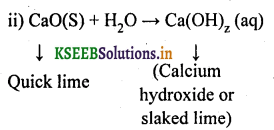Question 5.
Why is the amount of gas collected in one of the test tube in activity 1.7 double of the amount collected in the other? Name this gas.
Water contains two parts of hydrogen and one part of oxygen. Therefore during the electrolysis of water the amount of hydrogen gas collected in one of the test tubes is double than that of the oxygen produced and collected in the other test tube.

Question 6.
Why does the colour of copper sulphate solution change when an iron nail is dipped in it?
When an iron nail is dipped is copper sulphate solution, it becomes brownish in colour and wlour of copper sulphate fades due to the following reaction
Fe(s) + CuSO4 (aq) → FeSO4 (aq) + Cu
In this reaction, iron has replaced copper from its solution. Thus the amount of copper sulphate decreases and its colour fades.

Question 7.
Give an example of a double displacement reaction other than the one given in activity 1.10?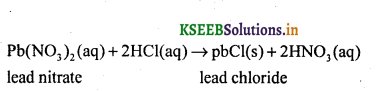Question 8.
Identity the substances that are oxidised & reduced in the following reactions
(i) 4Na(s) + O2 (g) → 2Na2O(s)
(ii) CuO(s) + H2 (g) → Cu(s) + H2O(I)
(i) Substances oxidised → Na(s), H2 (g)
(ii) Substances reduced → O2 (g), CuO(s)

### KSEEB Class 10 Science Chemical Reactions and Equations Textbook Exercise Questions and Answers

Question 1.
Which of the statements about the reaction below.are incorrect?
2PbO(s) + C(s) → 2Pb(s) + CO2(g)
(b) Carbon dioxide is getting oxidised.
(c) Carbon is getting oxidised.
(d) Lead oxide is getting reduced.
(i) (a) and (b)
(ii) (a) and (c)
(iii) (a), (b) and (c)
(iv) all
(i) (a) and (b)Question 2.
Fe2O3 + 2Al → Al2O3 + 2Fe
The above reaction is an example of a
(a) combination reaction
(b) double displacement reaction.
(c) decomposition reaction.
(d) displacement reaction
(d) displacement reaction.

Question 3.
What happens when dilute hydrochloric acid is added to iron fillings? Tick the correct answer.
(a) Hydrogen gas and iron chloride are produced.
(b) Chlorine gas and iron hydroxide are produced.
(c) No reaction takes place.
(d) Iron salt and water are produced.
(a) Hydrogen gas and iron chloride are produced.

Question 4.
What is balanced chemical equation? Why should chemical equations be in a balanced?
In a balanced equation, the number of atoms of each element on both sides of the equation are equal. A balanced equation satisfies mass balance as well as charge balance.

Question 5.
Translate the following statements into chemical equations and then balance them.
(a) Hydrogen gas combines with nitrogen to form ammonia.
(b) Hydrogen sulphide gas burns in air to give water and sulphur dioxide.
(c) Barium chloride reacts with aluminium sulphate to give aluminium chloride and a precipitate of barium sulphate.
(d) Potassium metal reacts with water to give potassium hydroxide and hydrogen gas.
(a) 3H2(g) + N2(g) → 2NH3(g)
(b) 2H2S(g) + 3O2(g) → 2SO2(g) + 2H2O(I)
(c) 3 BaCl2(aq) + Al2(SO4)3 (aq) → 2 AlCl3(aq) + 3 BaSO4(S).
(d) 2 K(s) + 2 H2O(l) → 2KOH (aq) + H2(g).

Question 6.
Balance the following chemical equations.
(a) HNO3 + Ca(OH)2 → Ca(NO3)2 + H2O
(b) NaOH + H2SO4 → Na2SO4 + H2O
(c) NaCl + AgNO3 → AgCl + NaNO3
(d) BaCl2 + H2SO4 → BaSO4 + HCl
(a) 2HNO3 + CA(OH)2 → Ca(NO3)2 + 2H2O
(b) 2NaOH + H2SO4 → Na2SO4 + 2H2O
(c) NaCl + AgNO3 → AgCl + NaNO3
(d) BaCl2 + H2SO4 → BaSO4 + 2HClQuestion 7.
Write the balanced chemical equations for the following reactions.

(a) Calcium, hydroxide + Carbon dioxide → Calcium carbonate + Water
(b) Zinc + Silver nitrate → Zinc nitrate + Silver
(c) Aluminium + Copper chloride → Aluminium chloride + Copper
(d) Barium chloride + Potassium sulphate + Barium sulphate + Potassium chloride.
(a) Ca (OH)2 + CO2 → CaCO3 + H2O
(b) Zn + 2 AgNO → Zn (NO3)2 + 2Ag
(c) 2 Al + 3 CuCl2 →2 AlCl3 + 3 Cu
(d) BaCl2 + K2SO4 → BaSO4 + 2KCl

Question 8.
Write the balanced chemical equation for the following and identify the type of reaction in each case.
(a) Potassium bromide(aq) + Barium iodide(aq) → Potassium iodide(aq) + Barium bromide(s)
(b) Zinc carbonate(s) → Zinc oxide(s) + Carbon dioxide(g)
(c) Hydrogen(g) + Chloride(g) → Hydrogen chloride(g)
(d) Magnesium(s) + Hydrochloric acid(aq)  → Magnesium chloride(aq) + Hydrogen(g)
(a) 2 KBr(aq) + Bal2(aq) → 2 KI(aq) + BaBr2(S)
Type →  Double displacement reaction

(b) ZnCO3(s) →  ZnO(s) + CO2(g)
Type → Decomposition reaction

(c) H2(g) + Cl2(g) → 2 HCl(g)
Type → Combination reaction

(d) Mg(s) + 2 HCl(aq) MgCl2(aq) + H2(g)
Type → displacement reaction

Question 9.
What does one mean by exothermic and endothermic reactions? Give examples.
Exothermic Reactions
Chemical reactions in which energy is evolved or given out are known as exothermic reactions.

• Ex: 1) C(s) + O2(g) → CO2(g) + 393.7 KJ (ΔH = -393.7 KJ)
• 2) N2(g) + 3H2(g) → 2NH3(g) + 92 KJ (ΔH = -92 KJ)

Endothermic reactions:
Reactions in which energy is absorbed are called endothermic reactions.

• Ex: 1) C(s) + 2S(s) → CS2(l) -92 KJ (ΔH = + 92 KJ)
• 2) N2(g) + O2(g) → 2NO(g) – 180.5 KJ (ΔH = + 180.5 KJ)

Question 10.
Why is respiration considered an exothermic reaction? Explain.
During digestion, food is broken down into simpler substances. Food like rice, potato and bread are made up of carbohydrates. These carbohydrates are further broken down to glucose. Glucose during respiration is oxidised with the liberation of energy as shown below.Thus, respiration is an exothermic process.

Question 11.
Why are decomposition reactions called the opposite of combination reactions? Write equations for these reactions.

• In a decomposition reaction, a single compound breaks down to produce two or more simpler substances.
Ex: 2HgO → 2Hg + O2
• In a combination reaction, two or more substance simply combine to form a new substance.
Ex: 2 Mg + O2 → 2 MgOQuestion 12.
Write, one equation each for decomposition reactions where energy is supplied in the form of heat, light or electricity.Question 13.
What is the difference between displacement and double displacement reactions? Write equations for these reactions.

 Displacement reaction Double displacement reaction 1. This is the reaction where one element has displaced or removed another element is known as displacement reaction. Reaction in which there is an exchange of ions between the reactants are called double displacement reactions. 2. A + Bx ➝ Ax + B A is more reactive than B CuSO4(aq) + Zn(s) ➝ ZnSO4(aq) + Cu(s) AB + CD ➝ AD + CB Na2SO4(aq) + BaCl2(aq) ➝ BaSO4(s) + 2NaCl(aq)

Question 14.
In the refining of silver, the recovery of silver from silver nitrate solution involved displacement by copper metal. Write down the reaction involved.Question 15.
What do you mean by a precipitation reaction? Explain by giving examples.
When two reactants in solution react and one or more of the products is insoluble or forms a precipitate, the reaction is called a precipitation reaction.
Ex: When a solution of iron chloride and ammonium hydroxide are mixed, a brown precipitate of iron hydroxide is formed.Question 16.
Explain the following in terms of gain or loss of oxygen with two examples each.
(a) Oxidation (b) Reduction
(a) Oxidation: A chemical reaction in which a substance gains oxygen is called oxidation.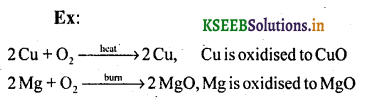(b) Reduction: A chemical reaction in which a substance loses oxygen is called reduction.
Ex: ZnO + C → Zn + CO, ZnO is reduced to Zn.
CuO + H2 → Cu + H2O, CuO is reduced to Cu

Question 17.
A shiny brown coloured element ‘X’ on heating in air becomes black in colour. Name the element ‘X’ and the black coloured compound formed.
Element X = Copper (Cu)
Compound = Copper oxide (CuO)
2Cu(s) + O2 → 2CuO
Brown (from air) BlackQuestion 18.
Why do we apply paint on iron articles?
Paint acts as antioxidant and saves iron articles from damage due to corrosion. It acts as buffer between iron article and moist air.

Question 19.
Oil and fat containing food items are flushed with nitrogen. Why?
To keep food items fresh and save these from oxidising effect of oxygen, food items are flushed with nitrogen.

Question 20.
Explain the following terms with one example each.
(a) Corrosion
(b) Rancidity
a) Corrosion:
When a metal is attacked by substances around it such as moisture, acids etc., it is said to corrode and this process is called corrosion.
Eq: The black coating on silver and the green coating on copper.

b) Rancidity:
When fats and oils are oxidised, they become rancid and their smell and taste change.
We can prevent Rancidity by following methods:

1.  Preserving food material in air tight container.
2.  We can preserve food items in Refrigirator.
4.  By Flushing with Nitrogen to prevent oxidation.

### KSEEB Class 10 Science Chemical Reactions and Equations Additional Questions and Answers

I. Fill in the blanks:

Question 1.
In an ionic equation, not only total number of atoms on both sides of equation should be ———-  but also —– on both sides should be equal.
equal, change

Question 2.
The balancing of chemical equation is in accordance with law of——— of mass.
ConservationQuestion 3.
—– reactions are usually / normally carried out in the presence of heat, light or electricity.
Decomposition

Question 4.
Electrons are lost and gained during
redox

II. Higher order Thinking Skill (HOTS) Questions:

Question 1.
Samuel had a silver coin which turned black. He kept the coin in the bowl lined with aluminium foil. Then he filled the bowl with water and boiled it. After some time, he found that the coin has become new. Its blackness disappeared. How did it happen?
The blackness of silver coin is due to the formation of silver sulphide on its surface due to its exposure to air on boiling, the aluminium foil reacts with the layer of silver sulphide and displaces silver from silver sulphide to form aluminium sulphide and silver. This makes the coin shiny.
3 Ag2S + 2 Al → 6 Ag + Al2S3

Question 2.
A substance ‘x’ displaces ‘y’ from its solution in water. It is called displacement reaction. What other chemical name can be given to such type of reactions’ explain giving an example?
Zinc displaces copper from its solution of copper sulphate.
Zn + CuSO4 → Cu + ZnSO4
It is also called redox reaction. Zn is oxidised to Zn2+ and Copper (Cu+2) is reduced to copper metal (Cu).

Question 3.
A student was given 5g of a pale green solid and was asked to observe what happens when it is heated. He found that on heating yellow coloured gas evoloved giving smell of burning sulphur and a reddish brown solid was left behind. When Zn is added to a solution of given compound, pale green colour fades away. Explain the above reaction?
A yellow coloured gas with smell of burning sulphur is given by oxides of sulphur further a pale green solid of sulphur compound is ferrous sulphate. This is further confirmed because pale green colour of ferrous sulphate fades away due to displacement reaction by adding Zn. Thus the above reaction areIII. Multiple Choice Questions:

Question 1.
Which one of the following changes is not oxidation?
(a) Combination
(b) Double displacement
(c) Corrosion
(d) Rancidity
(b) Double displacement

Question 2.
When copper Vessels are kept over a period of time, these get coated with a green surface. This is due to
(i) oxidation of copper
(ii) Corrosion of copper
(iii) reduction of copper
(iv) hydrolysis of copper
(a) (i) & (ii)
(b) (ii) & (iii)
(c) (i) & (iv)
(d) (ii) and (iv)
(a) (i) & (ii)

Question 3.
Which amongst the following does not hold good for a balanced chemical equation?
(a) Obeys law of conservation of energy and mass
(b) Has equal number of atoms of each element on both sides of the equation.
(c) Mass of reactants is equal to mass of the products.
(d) Has equal number of charges on both sides of the equation.
(a) Obeys law of conservation of energy and mass

Question 1.
Why is the combustion of LPG a chemical change?
Combustion of LPG is a chemical change because after its combustion the new substance formed changes 7. chemically and cannot be turned back into LPG.

Question 2.
Why is hydrogen peroxide kept in coloured bottles?
This is done in order to cut off light because hydrogen peroxide decomposes into water and oxygen in the presence of light.

Question 3.
Why is photosynthesis considered as an endothermic reaction?
Photosynthesis is an endothermic reaction because energy, in the form of sunlight is absorbed during the process of photosynthesis by green plants.Question 4.
What type of reaction is represented by the digestion of food in our body?
Decomposition reaction

Question 5.
Why do fireflies glow at night?
Fireflies have a protein which in the presence of an enzyme undergoes aerial oxidation. This is a chemical reaction which involves emission of visible light therefore fireflies glow at night.

Question 6.
Give reasons.
(a) Aluminium is a reactive metal but is still med for packing food articles.
On exposure to air, aluminium forms a hard protective layer of aluminium oxide (A1703) Which prevent further oxidation.

(b) Red litmus paper turns blue when touched with aqueous solution of magnesium oxidel
Magnesium oxide is an oxide of metal so, it is basic in nature. Due to its basic character it turns red litmus paper blue when touched with its aqueous solution.

Question 7.
Grapes hanging on the plants do not ferment but after being plucked from the plant can be fermented. Under what conditions do these grapes ferment? Is it a chemical or a physical change?
Grapes, when attached to the plants, are living and therefore, their own immune system prevents fermentation. The microbes can grow in the plucked grapes and under anaerobic condition, these can be fermented. This is a chemical change.

Question 8.
What is the role of a catalyst in a chemical reaction?
Catalyst changes (usually increase but some times decrease) the rate of a chemical reaction without it self being consumed in the reaction.

Question 9.
Name the type of chemical reactions
(i) CaO + H2O → Ca(OH)2
Combination reaction

(ii) 3BaCl2 + Al2(SO4)3 → 2AlCl3 +3BaSO4
Double displacement reaction

(iii)Decomposition reaction.

1. Balance the following chemical equations.

1. HNO3 + Ca(OH)2 → Ca(NO3)2 + H2O
2. NaOH + H2SO4 → Na2SO4 + H2O
3. NaCl + AgNO3 → AgCl + NaNO3
4. BaCl2 + H2SO4 → BaSO4 + HCI

1. 2HNO3 + Ca(OH)2 → Ca(NO3)2 + 2H2O
2. 2NaOH + H2SO4 Na2SO4 + 2H2O
3. NaCl + AgNO3 → AgCl + NaNO3
4. BaCl2 + H2SO4 → BaSO4 + 2HCl2. Fill in the blanks:

1. Magnesium + oxygen → __________
2. S + O2 → SO2 is a _______________ reactions.
3. Precipitation reaction produce __________  salts.
4. If a substance gains oxygen it is said to be__________ .

1. Magnesium oxide
2. Combination reactions
3. insoluble
4. oxidised

3. Match the Following

A – B

1. CuSO4   – (a) pale green
2. FeSO4  – (b) Blue
3. Na2SO4 – (c) white
4. BaCI2 – (d) Milky white

1. – (b) blue
2. – (a) pale green
3. – (d) Milky white
4. – (c) White

Question 4.
What is chemical reactions?
The Transformation of chemical substance into another chemical substance is known as chemical reaction.

Question 5.
What are the difference between physical change and chemical change give example.
Physical change

• It is a temporary change.
• No New substance are formed.

Ex:
Evaporation
Breaking of glass
Melting of Ice

Chemical change

• It is permanent change
• New substance are formed

Ex: Rusting of Iron
Clotting of blood
digestions of food
Burning of wood

Question 6.
Write the characteristics of chemical reactions.

• Change in state
• Evolutions of gas
• Change in temperature
• Evolution of soundQuestion 7.
What are the difference between Reactant and product?

 Reactant Product Substance which takes part chemical reactions New substance formed after a     chemical reactions.

Question 8.
Draw a neat Labelled diagram of Electrolysis of water.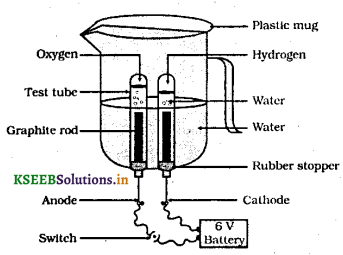Question 9.
On what chemical law balancing of chemical equation.
Balancing of a chemical equation is based on law of conservations of mass.

Question 10.
When do desert plants take up CO2 and perform photosynthesis.
In the desert plants the stomata are open at night they take CO2 at night and it is stored in the form of acid and it used during day time for photosynthesis.

Question 11.
Write the balanced chemical equation for the process of photosynthesis.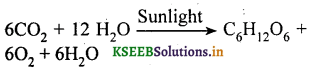Question 12.
When CO2 is passed through Limewater it turns Milky why?
Lime water(Ca(OH)2) combines with CO2 to form a suspension of CaCO3 which makes Lime water turn Milky.

Question 13.
Why are decomposition reactions called the opposite of combination reaction? Write the equation.
Decomposition reaction are those in which a compound breaks down to form two or more substance. This reaction required a sources of energy to produced. Thus they exact opposite of combination reaction in which two or more substance combines to give a new substance with the release of energy.
Ex: decomposition reaction
$$\mathrm{CaCO}_{3} \stackrel{\Delta}{\longrightarrow} \mathrm{CaO}+\mathrm{CO}_{2}$$
Combination reaction
CaO + H2O → Ca(OH)2 + Heat

Question 14.
Chemical equations of a combination reaction.

• 2Mg + O2 → 2MgO
• CaO + H2O → Ca(OH)2
• C + O2 → CO2
• S + O2 → SO2
• $$\mathrm{CaCO}_{3} \stackrel{\Delta}{\longrightarrow} \mathrm{CaO}+\mathrm{CO}_{2}$$
• NH3 + HCl → NH4Cl
• 2H2 + O2 → 2H2OQuestion 15.
Chemical equations of decomposition reactions.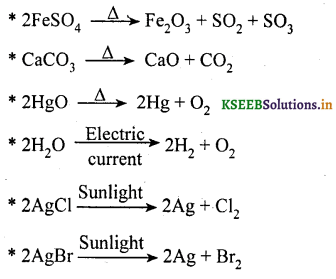Question 16.
Chemical equations of displacement reactions.

• Fe + CuSO4 → FeSO4 + Cu
• Zn + CuSO4 → ZnSO4 + Cu
• Pb + CuCl2 → PbCl2 + Cu

Question 17.
Chemical equations of double displacement reactions.